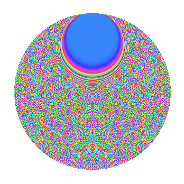# Properties

 Label 2009.2.bzLevel 2009 Weight 2 Character orbit bz Rep. character $$\chi_{2009}(8,\cdot)$$ Character field $$\Q(\zeta_{140})$$ Dimension 9312 Sturm bound 392

# Related objects

## Defining parameters

 Level: $$N$$ = $$2009 = 7^{2} \cdot 41$$ Weight: $$k$$ = $$2$$ Character orbit: $$[\chi]$$ = 2009.bz (of order $$140$$ and degree $$48$$) Character conductor: $$\operatorname{cond}(\chi)$$ = $$2009$$ Character field: $$\Q(\zeta_{140})$$ Sturm bound: $$392$$

## Dimensions

The following table gives the dimensions of various subspaces of $$M_{2}(2009, [\chi])$$.

Total New Old
Modular forms 9504 9504 0
Cusp forms 9312 9312 0
Eisenstein series 192 192 0

## Trace form

 $$9312q - 50q^{2} - 40q^{3} - 414q^{4} - 50q^{5} - 52q^{6} - 48q^{7} - 50q^{8} + O(q^{10})$$ $$9312q - 50q^{2} - 40q^{3} - 414q^{4} - 50q^{5} - 52q^{6} - 48q^{7} - 50q^{8} - 6q^{10} - 32q^{11} - 44q^{12} - 40q^{13} - 70q^{14} - 40q^{15} + 362q^{16} - 52q^{17} - 96q^{18} - 104q^{19} - 110q^{20} - 60q^{21} - 56q^{22} - 30q^{23} - 402q^{25} + 8q^{26} - 106q^{27} - 158q^{28} + 192q^{29} - 48q^{30} + 88q^{31} - 90q^{33} + 8q^{34} + 12q^{35} - 130q^{36} - 42q^{37} - 132q^{38} - 50q^{39} + 40q^{40} - 52q^{41} - 336q^{42} - 50q^{43} - 288q^{44} - 194q^{45} - 250q^{46} - 116q^{47} - 132q^{48} - 40q^{49} + 6q^{51} - 112q^{52} - 70q^{53} - 182q^{54} - 66q^{55} - 106q^{56} - 2q^{57} - 100q^{58} + 78q^{59} - 360q^{60} - 130q^{61} - 50q^{62} - 156q^{63} - 422q^{64} - 84q^{65} + 286q^{66} - 80q^{67} + 8q^{68} + 88q^{69} - 604q^{70} + 52q^{71} - 330q^{72} - 50q^{74} + 224q^{75} - 588q^{76} - 50q^{77} - 262q^{78} - 124q^{79} - 200q^{80} + 1624q^{81} + 192q^{82} - 232q^{83} - 40q^{84} - 28q^{85} + 2q^{86} - 50q^{87} + 190q^{88} - 172q^{89} + 70q^{90} - 154q^{92} + 48q^{93} + 6q^{94} - 18q^{95} + 524q^{96} + 100q^{97} - 66q^{98} - 220q^{99} + O(q^{100})$$

## Decomposition of $$S_{2}^{\mathrm{new}}(2009, [\chi])$$ into newform subspaces

The newforms in this space have not yet been added to the LMFDB.

## Hecke Characteristic Polynomials

There are no characteristic polynomials of Hecke operators in the database